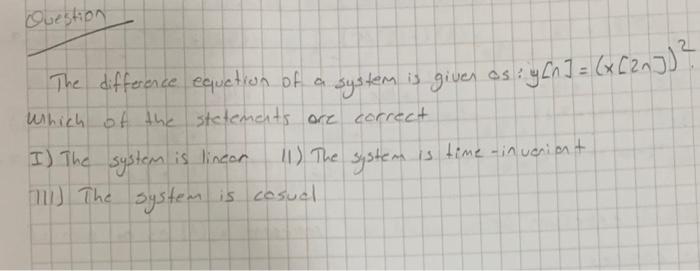Home / Expert Answers / Electrical Engineering / the-difference-equation-of-a-system-is-given-os-y-n-x-2-n-2-which-of-the-stetemats-or-pa846

# (Solved): The difference equation of a system is given os: \( y[n]=(x[2 n])^{2} \). Which of the stetemats or ...The difference equation of a system is given os: \( y[n]=(x[2 n])^{2} \). Which of the stetemats ore correct I) The system is lineor 11) The system is time-inuaiant 111) The system is cosuel

We have an Answer from Expert